# Check for NaN in Pandas DataFrame

NaN stands for Not A Number and is one of the common ways to represent the missing value in the data. It is a special floating-point value and cannot be converted to any other type than float. NaN value is one of the major problems in Data Analysis. It is very essential to deal with NaN in order to get the desired results.The ways to check for NaN in Pandas DataFrame are as follows:

• Check for NaN under a single DataFrame column:
• Count the NaN under a single DataFrame column:
• Check for NaN under the whole DataFrame:
• Count the NaN under the whole DataFrame:

Method 1: Using isnull().values.any() method
Example:

## Python3

 `# importing libraries ` `import` `pandas as pd ` `import` `numpy as np ` ` `  ` `  `num ``=` `{``'Integers'``: [``10``, ``15``, ``30``, ``40``, ``55``, np.nan, ` `                    ``75``, np.nan, ``90``, ``150``, np.nan]} ` ` `  `# Create the dataframe ` `df ``=` `pd.DataFrame(num, columns``=``[``'Integers'``]) ` ` `  `# Applying the method ` `check_nan ``=` `df[``'Integers'``].isnull().values.``any``() ` ` `  `# printing the result ` `print``(check_nan) `

Output: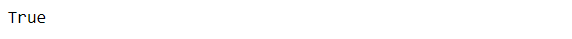It is also possible to to get the exact positions where NaN values are present. We can do so by removing .values.any() from isnull().values.any() .

## Python3

 `check_nan ``=` `df[``'Integers'``].isnull()`

Output: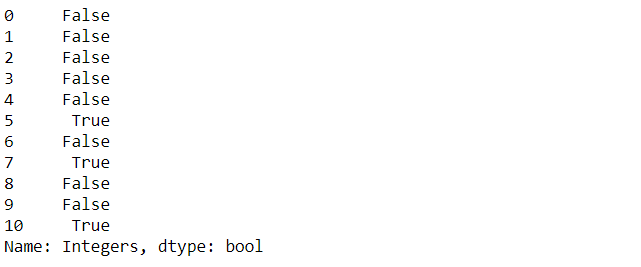Method 2: Using isnull().sum() Method
Example:

## Python3

 `# importing libraries ` `import` `pandas as pd ` `import` `numpy as np ` ` `  ` `  `num ``=` `{``'Integers'``: [``10``, ``15``, ``30``, ``40``, ``55``, np.nan, ` `                    ``75``, np.nan, ``90``, ``150``, np.nan]} ` ` `  `# Create the dataframe ` `df ``=` `pd.DataFrame(num, columns``=``[``'Integers'``]) ` ` `  `# applying the method ` `count_nan ``=` `df[``'Integers'``].isnull().``sum``() ` ` `  `# printing the number of values present ` `# in the column ` `print``(``'Number of NaN values present: '` `+` `str``(count_nan)) `

Output: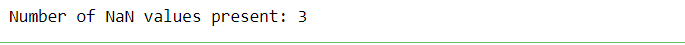Method 3: Using isnull().values.any() Method

Example:

## Python3

 `# importing libraries ` `import` `pandas as pd ` `import` `numpy as np ` ` `  `nums ``=` `{``'Integers_1'``: [``10``, ``15``, ``30``, ``40``, ``55``, np.nan, ``75``, ` `                       ``np.nan, ``90``, ``150``, np.nan], ` `        ``'Integers_2'``: [np.nan, ``21``, ``22``, ``23``, np.nan, ``24``, ``25``, ` `                       ``np.nan, ``26``, np.nan, np.nan]} ` ` `  `# Create the dataframe ` `df ``=` `pd.DataFrame(nums, columns``=``[``'Integers_1'``, ``'Integers_2'``]) ` ` `  `# applying the method ` `nan_in_df ``=` `df.isnull().values.``any``() ` ` `  `# Print the dataframe ` `print``(nan_in_df) `

Output: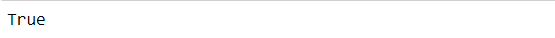To get the exact positions where NaN values are present, we can do so by removing .values.any() from isnull().values.any() .

Method 4: Using isnull().sum().sum() Method
Example:

## Python3

 `# importing libraries ` `import` `pandas as pd ` `import` `numpy as np ` ` `  `nums ``=` `{``'Integers_1'``: [``10``, ``15``, ``30``, ``40``, ``55``, np.nan, ``75``, ` `                       ``np.nan, ``90``, ``150``, np.nan], ` `        ``'Integers_2'``: [np.nan, ``21``, ``22``, ``23``, np.nan, ``24``, ``25``, ` `                       ``np.nan, ``26``, np.nan, np.nan]} ` ` `  `# Create the dataframe ` `df ``=` `pd.DataFrame(nums, columns``=``[``'Integers_1'``, ``'Integers_2'``]) ` ` `  `# applying the method ` `nan_in_df ``=` `df.isnull().``sum``().``sum``() ` ` `  `# printing the number of values present in ` `# the whole dataframe ` `print``(``'Number of NaN values present: '` `+` `str``(nan_in_df)) `

Output: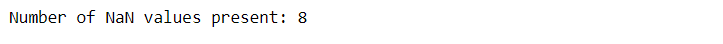My Personal Notes arrow_drop_upIf you like GeeksforGeeks and would like to contribute, you can also write an article using contribute.geeksforgeeks.org or mail your article to contribute@geeksforgeeks.org. See your article appearing on the GeeksforGeeks main page and help other Geeks.

Please Improve this article if you find anything incorrect by clicking on the "Improve Article" button below.

Article Tags :

8

Please write to us at contribute@geeksforgeeks.org to report any issue with the above content.